• 【1】全期望数学公式在基础概率论中是一块非常重要内容。 研究生阶段此段内容涉及科目有《随机过程分析-随机变量数字特征》/《数字通信-通信系统衰落信道性能分析》 由于信道噪声通常使用某些随机变量，所以在...
【1】全期望数学公式在基础概率论中是一块非常重要的内容。 研究生阶段此段内容涉及科目有《随机过程分析-随机变量的数字特征》/《数字通信-通信系统衰落信道性能分析》
由于信道噪声通常使用某些随机变量，所以在边界问题/尾部概率/截尾效应   都可能出现这货的身影，只看不懂不过瘾，还是弄明白其原理。
【2】全期望数学公式推导 注：自己脑袋瓜不够用，参考前人

全期望数学公式

E{E(g(X，Y))Y}=E(g(X，Y))E{E(g(X))Y}      =E(g(X))E{E(g(X，Y))Y}=E(g(X，Y))<!--//--><![CDATA[//><!--
\begin{alignat}{}
E\{ E(g(X，Y))Y \} =  E(g(X，Y)) \\
E\{ E(g(X))Y \} \ \ \ \ \ \ =  E(g(X)) \\
E\{ E(g(X，Y))Y \} =  E(g(X，Y))
\end{alignat}
//--><!]]>证： Take<!--//--><![CDATA[//><!--
Take \\
//--><!]]>S(y)=E[g(X,Y)|Y=y]=E[g(X,y)|y]==>E[g(X,Y)|Y)]=S(Y)                               ==>E[E(g(X,Y)|Y)]=E[S(Y)]                    ==>∫+∞−∞S(y)dFY(y)=∫+∞−∞E[g(X,y)|y]dFY(y)==>∫+∞−∞[∫+∞−∞g(x,y)dFx|y(x|y)]dFY(y)  ==>∫+∞−∞∫+∞−∞g(x,y)[dFx|y(x|y)dFY(y)]  ==><!--//--><![CDATA[//><!--
\begin{alignat}{}
S(y)=E\left[ g(X,Y)|Y=y\right]=E\left[g(X,y)|y\right]==> \\
E\left[g(X,Y)|Y)\right]=S(Y) \ \ \ \ \ \ \ \ \ \ \ \ \ \ \ \ \ \ \ \ \ \ \ \ \ \ \ \ \ \ \ ==>  \\
E\left[E(g(X,Y)|Y)\right]=E\left[S(Y)\right]  \ \ \ \ \ \ \ \ \ \ \ \ \ \ \ \ \ \ \ \ ==>\\
\int^{+\infty}_{-\infty}S(y)dF_Y(y)= \int^{+\infty}_{-\infty}E\left[ g(X,y)|y\right] dF_Y(y)==>\\
\int^{+\infty}_{-\infty} \left[ \int^{+\infty}_{-\infty}g(x,y)dF_{x|y}(x|y)  \right] dF_Y(y)  \ \ ==> \\
\int^{+\infty}_{-\infty}  \int^{+\infty}_{-\infty}g(x,y)\left[dF_{x|y}(x|y)   dF_Y(y)\right]  \ \ ==> \\
\end{alignat}
//--><!]]>due  to  F(x,y)=FY(y)Fx|y(x|y),so<!--//--><![CDATA[//><!--
due \ \ to \ \ F(x,y)=F_Y(y)F_{x|y}(x|y),so
//--><!]]>E[E(g(X,Y)|Y)]=E[S(Y)]=∫+∞−∞∫+∞−∞g(x,y)dF(x,y)=E[g(X,Y)]<!--//--><![CDATA[//><!--
\begin{alignat}{}
E\left[E(g(X,Y)|Y)\right]=E\left[S(Y)\right]  =\int^{+\infty}_{-\infty}  \int^{+\infty}_{-\infty}g(x,y)dF(x,y)=E \left[g(X,Y) \right]
\end{alignat}
//--><!]]>描述

变量的定义不同，可得到剩下两个式子，强盗逻辑，按照你想要的结果预定义。
展开全文• 如果只是简单看看条件随机场和最大熵模型...之前看条件随机场的时候计算特征函数模型期望是下面这样的公式: 计算先验模型期望时看到这样的公式： 式子中有先验分布，实现算法时，p(x)和p(x,y)对于先验分布的遍...
如果只是简单看看条件随机场和最大熵模型的原理。我打赌绝大多数人没有意识到我下面提到的公式是这个意思。自己实现算法涉及到细节时稀里糊涂写出来，最近看到一个最大熵模型的实现想不通的时候，才慢慢想明白为什么这样写。

正文：

之前看条件随机场的时候计算特征函数模型期望是下面这样的公式: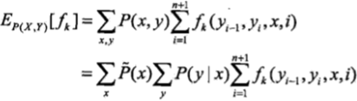计算先验模型期望时看到这样的公式：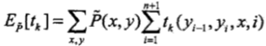式子中有先验分布，实现算法时，p(x)和p(x,y)对于先验分布的遍历我一直很懵逼，到底这里的x和y是序列的遍历还是x概率分布或者x和y的概率分布的遍历。以至于之前我写的时候默认就写的对于序列的遍历。

但回想起概率论中求期望这个过程一定是在一个概率分布上进行的啊。

比如：

A

X

0

0.2

1

0.1

2

0.3

3

0.4

计算A在随机变量X上的期望,毫无疑问:

E = 0*0.2 + 1*0.1 + 2 * 0.3 + 3 * 0.4 = 1.9

所以一定要相信科学啊！！！上面的式子中也是这样的，只不过我们可以把这个过程在整个训练数据序列中进行。下面以最大熵模型中求特征函数期望为例来解释。

最大熵模型中，求特征函数模型期望公式如下：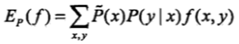求先验期望如下：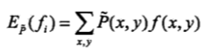先说先验期望：

看下面一段代码：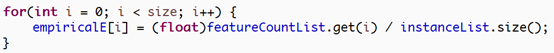这里empiricalE就是先验期望，i是遍历所有特征函数，featureCountList.get(i)指的是特征函数i在训练数据中出现的次数。InstanceList.size()是样本的总个数。注意到特征函数由一个x和y来决定。这里你可以看到，featureCountList.get(i) / instanceList.size()不就是求了一个p(x,y)的概率值吗？怎么就成了期望了？

再次想想上面说的求期望过程：

我们知道p(x,y)这个分布中x，y的值和特征函数中x0和y0的值(假设这个特征函数中的x和y是x0和y0)完全对应的只有一个。那就是p(x0,y0)而特征函数的值又为1，所以就是一个概率值p(x0,y0)了。

也就是说这个求期望的过程是这样的：

E = 1 * p(x0,y0) + 0 *p(x1,y1)+….

_再看看这个求先验期望的公式：这里对x和y的遍历是再p(x,y)分布上，而非序列上进行的。

再来看看求模型期望的过程：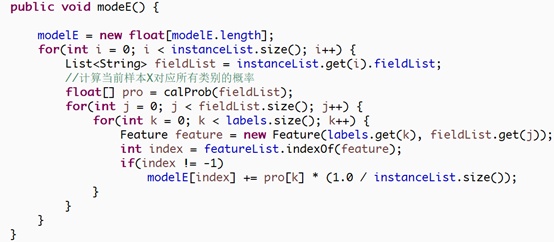对照公式:先解释一下，每个instance就是一个样本，而fieldList就是一个样本中的所有特征值，比如x1，x2，x3…

calProb就是计算公式中的p(y|x), Feature是特征函数，featureList是特征函数集合。

仔细看看，最外层的循环是整个序列的遍历，第2层是对一个样本中的特征数的遍历，这2层合起来就是对x的遍历，最内层的是对标签y的遍历。

这里的x是整个序列。最内层判断如果该特征函数存在就累加对应特征函数的期望值，看到里面的计算可能懵了，pro[k] * 1/instanceList.size(),先验概率p(x)跑哪里去了？？

实际上这里的累加隐含了求先验概率p(x)。

假设某个x0的先验概率是1/5，这里只不过加了很多次，最后累积起来还是1/5。

还有值得注意的一点是：这里的p(y|x)中x是单个样本中的所有特征。我们这里求模型的期望依然是特征函数关于p(x,y)的期望。注意因为x是多维度，这里假设有2个维度，那么

P(x,y) = p(x1,x2,y) = p(x1,x2) * p(y|x1,x2),这里的p(x1.x2)就是先验概率了，求特征函数f(x,y)关于p(x1,x2,y)的期望，需要注意最大熵中，特征函数中的x是单个维度的，比如现在求的是f(x1=a,y=b)关于p(x1,x2,y)的期望。我们不关心先验p(x1,x2)，只要这个样本的x1=a并且y=b，那么特征函数就为1，先验概率自然会被累积。我们能在分布先对x，y遍历吗，那样应该简单多了。当然不行，那样就变成先验分布，我们要的就是根据模型参数来计算期望。最后尽可能的优化到使模型期望和先验期望一样。

2019.8.8

补充解释：因为求模型期望并不能直接在p(x,y)上直接进行，那样就成了先验期望，所以必须在序列上完成求模型的期望，和求先验期望相比，我们并不能直接得到先验p(x=a)，而是在序列中的哪些出现x=a的位置上去每次单个计算这个先验，比如序列长度为5，在位置1，4出现了a，就相当于每次出现a时累加一次1/5，那么共出现了2次，最后也会得到2/5，这个和先验期望中的p(x)概率是一样的.

对同一特征函数求期望，pro[k]中的k都是一样的，k是标签y，pro[k]是p(y|x),但是区别于样本又不太一样，因为p(y|x)计算的是p(y|x1,x2,x3...)。特征函数只关乎某个维度的x和一个标签y。这里举的例子只是好直白的解释，你也可以理解为每出现这样一个特征函数，对模型期望就贡献1/instanceList.size()，只是不能这么直白就说是相加，最后优化的目标是调整特征函数权重使得模型期望尽可能和先验期望一样。某个特征函数先验期望是2/5，那么模型期望就是1/5*a+1/5*b

感谢观看，觉得不错的话可以考虑支持下我，用我的AI大师码0415在滴滴云上购买GPU/vGPU/机器学习产品可享受9折优惠，点击www.didiyun.com前往滴滴云官网。
GPU：https://www.didiyun.com/production/gpu.html
VGPU：https://www.didiyun.com/production/vgpu.html
机器学习工作室（DAI）：https://www.didiyun.com/production/dai.html

展开全文CRF
• 条件均值与全期望公式】   假定两个连续随机变量X,YX,YX,Y，它们联合概率密度为 pX,Y(x,y)=pX(x)pY∣X(y∣x)=pY(y)pX∣Y(x∣y)p_{\rm X,Y}(x,y)=p_{\rm X}(x)p_{\rm Y|X}(y|x)=p_{\rm Y}(y)p_{\rm X|Y}(x|y)...
【条件均值与全期望公式】
假定两个连续的随机变量$X,Y$，它们的联合概率密度为
$p_{\rm X,Y}(x,y)=p_{\rm X}(x)p_{\rm Y|X}(y|x)=p_{\rm Y}(y)p_{\rm X|Y}(x|y)$则有条件均值${\mathbb E}[X|Y=y]={\mathbb E}[X|y]$为
${\mathbb E}[X|y]=\int_{-\infty}^{\infty}xp_{\rm X|Y}(x|y)dx=\int_{-\infty}^{\infty}x\frac{p_{\rm X,Y}(x,y)}{p_{\rm Y}(y)}dx.$进一步我们来推导全期望数学公式
${\mathbb E}_{\rm Y}\{{\mathbb E}_{\rm X|Y}[X|Y]\}={\mathbb E}_{\rm X}[x].$证明如下：
${\mathbb E}_{\rm Y}\{{\mathbb E}_{\rm X|Y}[X|Y]\}=\int_{-\infty}^{\infty}\{{\mathbb E}_{\rm X|Y}[X|y]\}p_{\rm Y}(y)dy$$=\int_{-\infty}^{\infty}[\int_{-\infty}^{\infty}xp_{\rm X|Y}(x|y)dx]p_{\rm Y}(y)dy$$=\int_{-\infty}^{\infty}x\int_{-\infty}^{\infty}p_{\rm X|Y}(x|y)p_{\rm Y}(y)dydx$$=\int_{-\infty}^{\infty}x[\int_{-\infty}^{\infty}p_{\rm X,Y}(x,y)dy]dx$$=\int_{-\infty}^{\infty}xp_{\rm X}(x)dx={\mathbb E}_{\rm X}(x)$


展开全文• Introduction 泊松分布由二项分布演进而来。 二项分布即，假设硬币正面向上概率为p，抛n次硬币，这n次中硬币朝上k次（k）概率为 p(k)=Cknpk(1−p)n−k (1) p(k) = C_n^kp^k(1-p)^{...硬币朝上的期望值为： E(k)=pn
Introduction

泊松分布由二项分布演进而来。
二项分布即，假设硬币正面向上概率为p，抛n次硬币，这n次中硬币朝上k次（k<=n）的概率为
p(k)=Cknpk(1−p)n−k            (1) p(k) = C_n^kp^k(1-p)^{n-k} \ \ \ \ \ \ \ \ \ \ \ \ (1)
硬币朝上的期望值为：
E(k)=pn                                  (2) E(k) = pn \ \ \ \ \ \ \ \ \ \ \ \ \ \ \ \ \  \ \ \  \ \ \ \ \ \ \ \ \ \ \ \ \  \ (2)
如果我们把期望值看做一个恒值 λ $\ \lambda$/extract_itex]。即：现在我能根据n的大小来控制 p $\ p \$，即 n $\ n \$越大， p $\ p\$越小，硬币朝上的次数的期望不变(恒为 λ $\ \lambda\$）： E(k)=pn=λ (3) E(k) = pn= \lambda \ \ \ \ \ \ \ \ \ \ \ \ \ \ \ \ \ \ \ \ \ \ \ \ \ \ \ (3) OK，引子到此结束，下面开始正式推导！！！ Derivation of Poisson distribution ⨂12⨂34$\sideset{^1_2}{^3_4}\bigotimes$⨂12⨂34$\sideset{^1_2}{^3_4}\bigotimes$⨂12⨂34$\sideset{^1_2}{^3_4}\bigotimes$⨂12⨂34$\sideset{^1_2}{^3_4}\bigotimes$⨂12⨂34$\sideset{^1_2}{^3_4}\bigotimes$⨂12⨂34$\sideset{^1_2}{^3_4}\bigotimes$⨂12⨂34$\sideset{^1_2}{^3_4}\bigotimes$⨂12⨂34$\sideset{^1_2}{^3_4}\bigotimes$⨂12⨂34$\sideset{^1_2}{^3_4}\bigotimes$⨂12⨂34$\sideset{^1_2}{^3_4}\bigotimes$⨂12⨂34$\sideset{^1_2}{^3_4}\bigotimes$⨂12⨂34$\sideset{^1_2}{^3_4}\bigotimes$⨂12⨂34$\sideset{^1_2}{^3_4}\bigotimes$⨂12⨂34$\sideset{^1_2}{^3_4}\bigotimes$⨂12⨂34$\sideset{^1_2}{^3_4}\bigotimes$⨂12⨂34$\sideset{^1_2}{^3_4}\bigotimes$⨂12⨂34$\sideset{^1_2}{^3_4}\bigotimes$⨂12⨂34$\sideset{^1_2}{^3_4}\bigotimes$ limn−>∞,p−>0=Cknpk(1−p)n−k (4) \ \ \ \ \lim_{n->\infty,p->0}=C_n^kp^k(1-p)^{n-k} \ \ \ \ \ \ \ \ \ \ \ \ \ \ \ \ \ \ \ \ \ \ \ \ \ \ (4) =limn−>∞,p−>0n!k!(n−k)!pk(1−p)n−k (5) =\lim_{n->\infty,p->0}\frac{n!}{k!(n-k)!}p^k(1-p)^{n-k} \ \ \ \ \ \ \ \ \ \ \ \ \ \ \ \ \ \ \ \ (5) =limn−>∞,p−>0n(n−1)...[n−(k−1)]k!pk(1−p)n−k (6) =\lim_{n->\infty,p->0}\frac{n(n-1)...[n-(k-1)]}{k!}p^k(1-p)^{n-k}\ \ (6) =limn−>∞,p−>0nkk!(λn)k(1−p)λp−k (7) =\lim_{n->\infty,p->0}\frac{n^k}{k!}(\frac{\lambda}{n})^k(1-p)^{\frac{\lambda}{p}-k} \ \ \ \ \ \ \ \ \ \ \ \ \ \ \ \ \ \ \ \ \ \ \ \ \ \ \ \ \ \ \ \ (7) =limn−>∞,p−>0nkn!λkk![(1−p)−1p]−λ(11−p)k (8) =\lim_{n->\infty,p->0}\frac{n^k}{n!}\frac{{\lambda}^k}{k!}[(1-p)^{-\frac{1}{p}}]^{-\lambda}(\frac{1}{1-p})^k \ \ \ \ \ \ \ \ \ \ \ \ \ \ \ (8) =limn−>∞,p−>01λkk!(1−λn)n(1−λn)−k (9) =\lim_{n->\infty,p->0}1\frac{{\lambda}^k}{k!}(1-\frac{\lambda}{n})^{n}({1-\frac{\lambda}{n})^{-k}} \ \ \ \ \ \ \ \ \ \ \ \ \ \ \ \ \ \ \ \ \ \ \ \ (9) =limn−>∞,p−>01λkk!(1−λn)n1 (10) =\lim_{n->\infty,p->0}1\frac{{\lambda}^k}{k!}(1-\frac{\lambda}{n})^{n}1 \ \ \ \ \ \ \ \ \ \ \ \ \ \ \ \ \ \ \ \ \ \ \ \ \ \ \ \ \ \ \ \ \ \ \ \ \ \ \ (10) - 回顾 e−λ $\ e^{-\lambda} \$的定义： - limn−>∞(1−λn)n=e−λ\lim_{n->\infty}(1-\frac{\lambda}{n})^n = e^{-\lambda} - - then,formula（10）can be rewrite as: =limn−>∞,p−>0λkk!e−λ (11) =\lim_{n->\infty,p->0}\frac{\lambda^k}{k!}e^{-\lambda}\ \ \ \ \ \ \ \ \ \ \ \ \ \ \ \ \ \ \ \ \ \ \ \ \ \ \ \ \ \ \ \ \ \ \ \ \ \ \ \ \ \ \ \ \ \ \ \ \ \ (11) ⨂12⨂34$\sideset{^1_2}{^3_4}\bigotimes$⨂12⨂34$\sideset{^1_2}{^3_4}\bigotimes$⨂12⨂34$\sideset{^1_2}{^3_4}\bigotimes$⨂12⨂34$\sideset{^1_2}{^3_4}\bigotimes$⨂12⨂34$\sideset{^1_2}{^3_4}\bigotimes$⨂12⨂34$\sideset{^1_2}{^3_4}\bigotimes$⨂12⨂34$\sideset{^1_2}{^3_4}\bigotimes$⨂12⨂34$\sideset{^1_2}{^3_4}\bigotimes$⨂12⨂34$\sideset{^1_2}{^3_4}\bigotimes$⨂12⨂34$\sideset{^1_2}{^3_4}\bigotimes$⨂12⨂34$\sideset{^1_2}{^3_4}\bigotimes$⨂12⨂34$\sideset{^1_2}{^3_4}\bigotimes$⨂12⨂34$\sideset{^1_2}{^3_4}\bigotimes$⨂12⨂34$\sideset{^1_2}{^3_4}\bigotimes$⨂12⨂34$\sideset{^1_2}{^3_4}\bigotimes$⨂12⨂34$\sideset{^1_2}{^3_4}\bigotimes$⨂12⨂34$\sideset{^1_2}{^3_4}\bigotimes$⨂12⨂34$\sideset{^1_2}{^3_4}\bigotimes$ 展开全文• UA MATH563 概率论的数学基础 鞅论初步2 条件期望的应用：推导二元随机变量的相关计算公式机器学习 • 为减小传统子集模拟法求解失效概率及可靠性灵敏度估计值的方差,提出了一种基于条件期望的子集模拟方法,其理论基础是全方差公式,即多维变量条件期望的方差不大于变量自身的方差。所提方法将传统子集模拟法中计算失效... • 不严格引入，随机变量X在事件B上的条件期望为： E(X∣B)=∫BXdPP(B), E(X|B)=\frac{\int_BXdP}{P(B)}, E(X∣B)=P(B)∫B​XdP​, 可以得到公式1： ∫BE(X∣B)dP=∫B∫BXdPP(B)dP=∫BXdPP(B)P(B)=∫BXdP \int_B E(X|... • 此处，给出条件熵（）定义:X给定条件下Y的条件概率分布熵对X数学期望： 在书中P61页下方给出了各类定义： 设训练数据集为D，表示样本容量，即样本个数，设有K个类，。为属于类样本个数，，设特征A有n...决策树 • 初见安~~~又开启数论探索啦~~：） 一。概率 1.基本定义 在概率论中，我们把一个随机事件一个可能结果称为其样本点，其所有样本点构成集合称之为样本空间。（注意，随机事件并不一定只有一种可能结果）在... • 注意伯努利分布关注是结果只有0和1，而不管观测条件是什么。 推导过程： 注：就是一次实验下结果。不是0就是1. 二：二项分布 本质： 就是n次实验下伯努利分布。 期望和方差 三：泊松分布 1.引入 很多... • 官方题解： 补充这个dp转移 概率系数 p(n−2)∗...由于人数是无限，题目告诉我们随机抽取一个号码概率是 1n\frac{1}{n}n1​，实际上是告诉我们每个位置分配概率是等可能。 枚举 A国人坐在第 i 个位置进行...贝叶斯 • 基于条件概率思想，在连续值命题逻辑系统中引入赋值密度函数概念，给出了公式的概率真度、数学期望条件概率真度定义，并得到了一些概率真度推理规则。证明了Lukasiewicz逻辑系统中概率真度、条件概率真度在... • 相信你对变量这个概念并不陌生，数学方程式和编程代码里经常会用到变量。那什么是变量呢？我们在概率中常说随机变量（ random variable）和普通变量（variable）又有什么... • 首先回忆一下条件概率的公式P(a|b) = P(ab) / P(b)http://blog.csdn.net/sdj222555/article/details/43025131代码参考来源 首先证明了最后的答案与每个骰子分别的情况无关 也就是每个骰子的情况可以在合法的范围内... • 用于 Var-of-CE 的不同基于方差分析的估计器的公式，发布为 IIM Kozhikode 工作论文 IIMK/WPS/338/ITS/2019/02，称为基于 n_k 的 Var-of-CE 估计器 - 它似乎具有不需要使用试点模拟计算 n* 的优点。 该脚本迭代调用...matlab • 从事件型全概公式出发,通过条件期望的定义及其重要性质推导出关于连续型随机变量的全概公 式形式,并给出它在概率计算中的应用 。 • 利用ITO公式和级数展开建立了最优投资决策问题的期望效益一个数学表示，并由此给出了Merton关于最优投资决策问题一个重要结果简化证明，同时还给出了一个判断投资决策问题最优停止时刻充分条件 • 题意：给出一颗n个点，n-1条边树，每个...然后考虑换根，建议画图理解，主要思想是将父节点中贡献排除这个点贡献（在这里是个条件概率公式），然后这个点依赖是它子树和父节点连接那一坨贡献。 Latex太烦了 • C - One Person Game ZOJ - 3329 转自一篇写非常好博客： ... 只需按照公式去求解A，B即可，我代码中dp数组只是预处理了一下个各个分数概率。 #include&lt;iostream&gt; #inc... • 在有些时候，直接计算随机变量的方差非常麻烦，此时可以用方差分解公式，将方差分解为条件期望的方差加条件方差的期望： Var(X)=Var[E(X∣Y)]+E[Var(X∣Y)] \text{Var}(X)=\text{Var}[\text{E}(X|Y)]+\text{E}[\text...数学 • 一旦实现这一目标，就可以期望自动O（a）改进机制在SF中起作用，这与SF标准公式形成了鲜明对比。 预期这将显着提高某些复合算子逐步缩放功能可达到精度。 此外，χSF提供了确定传统上从手性Ward身份获得... • 然后期望的几点性质: 对于数学期望，我们还应该明确一些知识点： (1) 期望的“线性”性质 对于所有满足条件的离散型的随机变量$$X,Y$$和常量$$a,b$$有: \[E(aX+bY)=aE(x)+bE(y)$ 即常说的"期望的和等于和的期望" ...
• 首先看条件的公式 定义：X给定条件下Y的条件概率分布的熵对X的数学期望 几点理解： X和Y并不一定是独立分布 给定条件下：这里的给定条件下是指，如果X确定的话，YY的条件概率分布的熵对X的数学期望，并不指X确定...机器学习 决策树
• 目录 随机事件与概率 1.充要条件； 2.条件概率： 3. 全概率公式 4.贝叶斯公式 随机变量及其分布 ...1. 随机变量 2....4. 数学期望: 5 期望的数学性质： 6. 方差： 7 期望的数学性质： 8 标准差 9...
• Expectation Maximization(EM) 不要强求不可知，要从已知推未知 在刚开始学习时，我觉得EM算法真难，反反复复就是...利用已知条件逐步探索未知，就好像玩mine craft，下矿时候火把总是从矿坑入口渐渐点亮整个...自然语言处理 数据挖掘 机器学习
• 上一节详解的阐述了条件随机场的定义和简单的学习算法推倒，这里不懂的前翻看前两节的博客，这里不再赘述，本节将主要求期望的问题，为什么要求解期望？本节主要参考的内容是一篇论文和李航的书，论文是...
• 我们的条件定义是：定义为X给定条件下，Y的条件概率分布熵对X数学期望 这个还是比较抽象，下面我们解释一下： 设有随机变量（X,Y），其联合概率分布为 条件熵H（Y|X）表示在已知随机变量X......